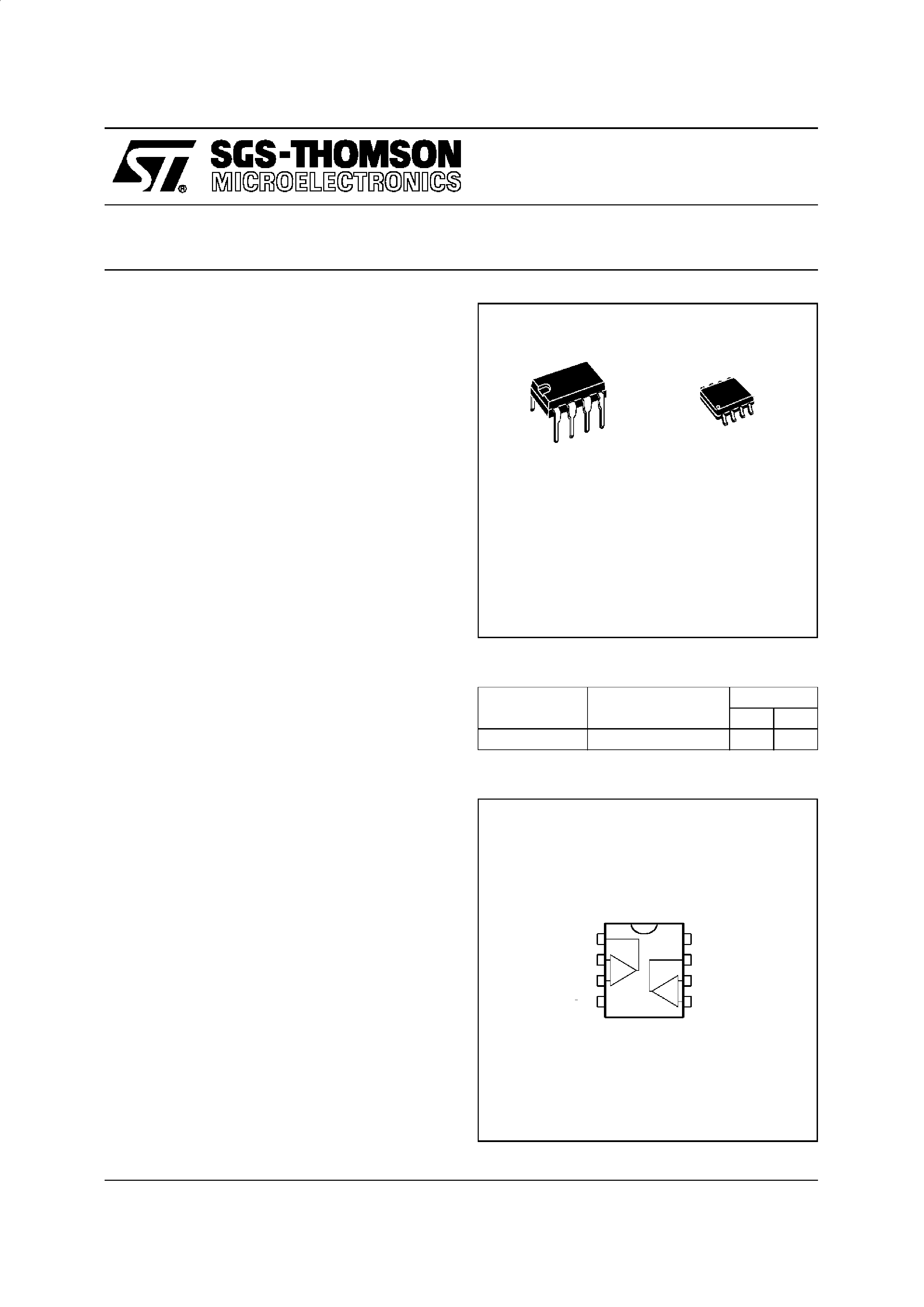TS3V912
3V INPUT/OUTPUT RAIL TO RAIL
DUAL CMOS OPERATIONAL AMPLIFIER
March 1996
.
DEDICATED TO 3.3V OR BATTERY SUPPLY
(specified at 3V and 5V)
.
RAIL TO RAIL INPUT AND OUTPUT
VOLTAGE RANGES
.
SINGLE SUPPLY OPERATION FROM 2.7V
TO 16V
.
EXTREMELY LOW INPUT BIAS CURRENT :
1pA TYP
.
LOW INPUT OFFSET VOLTAGE :
2mV max.
.
SPECIFIED FOR 600
.
LOW SUPPLY CURRENT : 200
µA/Ampli
(VCC = 3V)
.
ESD TOLERANCE : 3KV
.
LATCH-UP IMMUNITY
1
2
3
45
6
7
8
-
+
-
+
Inverting Input 1
Output 1
Non-inverting Input 1
VCC
VCC +
Output 2
Inverting Input 2
Non-inverting Input 2
PIN CONNECTIONS (top view)
DESCRIPTION
The TS3V912 is a RAIL TO RAIL dual CMOS
operational amplifier designed to operate with a
single 3V supply voltage.
The input voltage range Vicm includes the two
supply rails VCC
+ and VCC-.
The output reaches :
· V
CC
- +50mV
VCC
+ -50mV
with RL = 10k
· V
CC
- +350mV VCC+ -350mV with RL = 600
This product offers a broad supply voltage operat-
ing range from 2.7V to 16V and a supply current of
only 200
µA/amp. (VCC = 3V).
Source and sink output current capability is typi-
cally 40mA (at VCC = 3V), fixed by an internal
limitation circuit.
ORDER CODES
Part Number
Temperature Range
Package
ND
TS3V912I/AI/BI
-40, +125
oC
··
N
DIP8
(Plastic Package)
D
SO8
(Plastic Micropackage)
1/9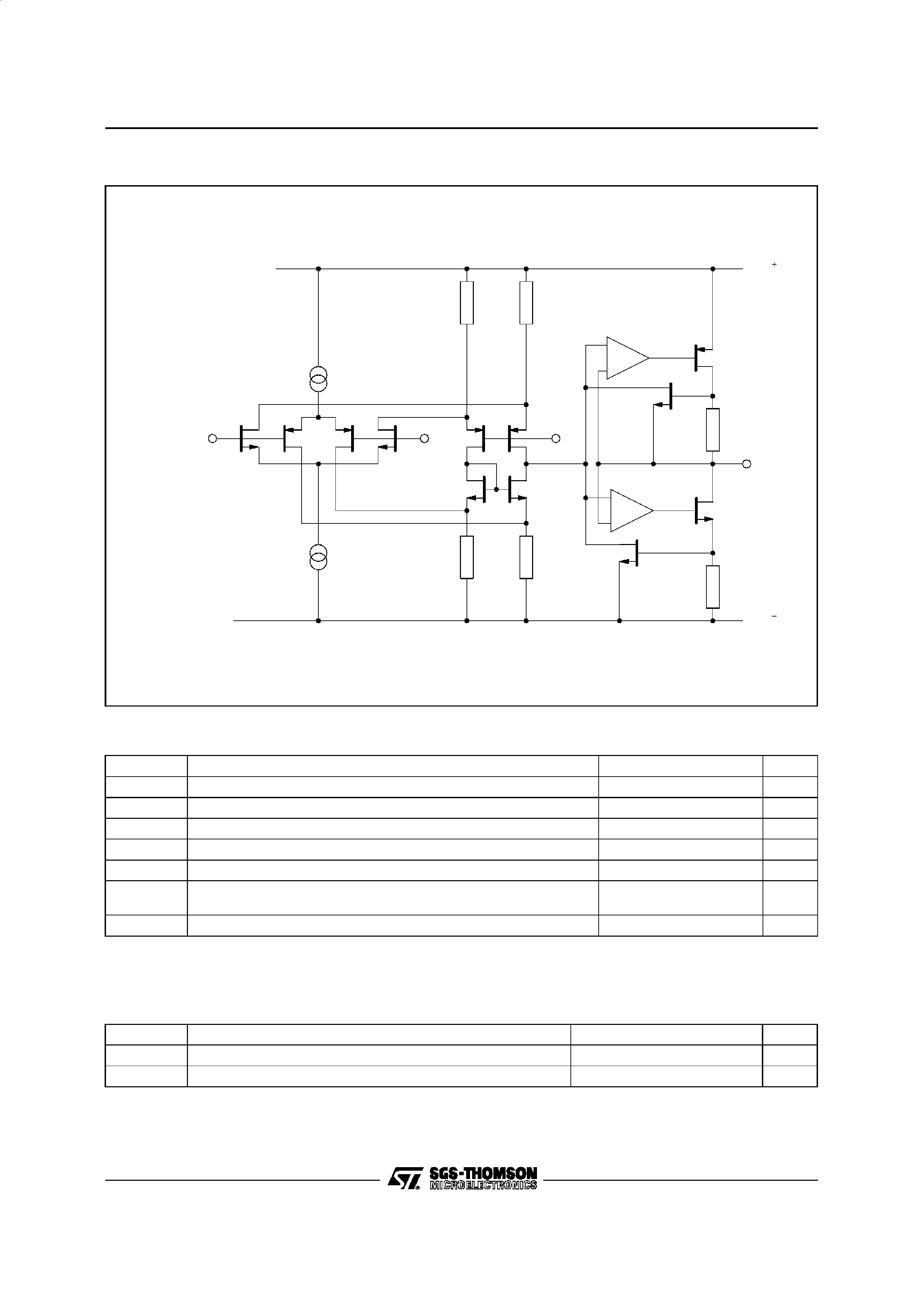ABSOLUTE MAXIMUM RATINGS
Symbol
Parameter
Value
Unit
VCC
Supply Voltage - (note 1)
18
V
Vid
Differential Input Voltage - (note 2)
±18
V
Vi
Input Voltage - (note 3)
-0.3 to 18
V
Iin
Current on Inputs
±50
mA
Io
Current on Outputs
±130
mA
Toper
Operating Free Air Temperature Range
TS3V912I/AI/BI
-40 to +125
oC
Tstg
Storage Temperature
-65 to +150
oC
Notes :
1. All voltage values, except differential voltage are with respect to network ground terminal.
2. Differential voltages are the non-inverting input terminal with respect to the inverting input terminal.
3. The magnitude of input and output voltages must never exceed VCC
+ +0.3V.
OPERATING CONDITIONS
Symbol
Parameter
Value
Unit
VCC
Supply Voltage
2.7 to 16
V
Vicm
Common Mode Input Voltage Range
VCC
- -0.2 to VCC++0.2
V
Non-inverting
Input
Inverting
Input
Inte rnal
Vre f
Output
VCC
VCC
SCHEMATIC DIAGRAM (1/2 TS3V912)
TS3V912
2/9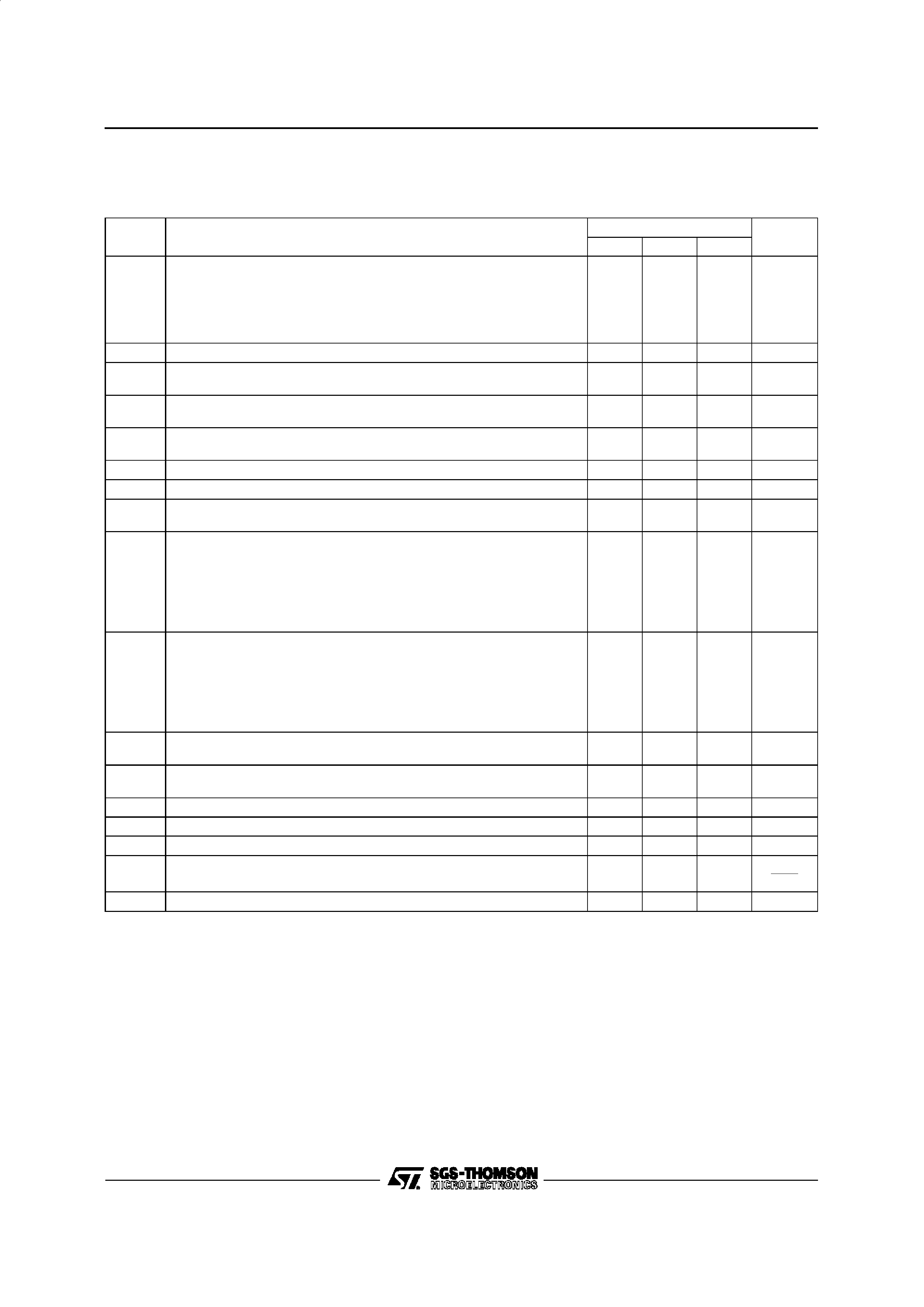ELECTRICAL CHARACTERISTICS
VCC
+ =3V, VCC- =0V, RL,CL connectedto VCC/2, Tamb =25oC (unless otherwise specified)
Symbol
Parameter
TS3V912I/AI/BI
Unit
Min.
Typ.
Max.
Vio
Input Offset Voltage (Vic =Vo =VCC/2)
TS3V912
TS3V912A
TS3V912B
Tmin.
Tamb Tmax.
TS3V912
TS3V912A
TS3V912B
10
5
2
12
7
3
mV
DVio
Input Offset Voltage Drift
5
µV/oC
Iio
Input Offset Current - (note 1)
Tmin.
Tamb Tmax.
1
100
200
pA
Iib
Input Bias Current - (note 1)
Tmin.
Tamb Tmax.
1
150
300
pA
ICC
Supply Current (per amplifier, AVCL = 1, no load)
Tmin.
Tamb Tmax.
200
300
400
µA
CMR
Common Mode Rejection Ratio
Vic = 0 to 3V, Vo = 1.5V
50
80
dB
SVR
Supply Voltage Rejection Ratio (VCC
+ = 2.7 to 3.3V, VO =VCC /2)
50
80
dB
Avd
Large Signal Voltage Gain (RL = 10k
,VO = 1.2V to 1.8V)
Tmin.
Tamb Tmax.
3
3
10
V/mV
VOH
High Level Output Voltage (Vid = 1V)
RL = 100k
RL = 10k
RL = 600
RL = 100
Tmin.
Tamb Tmax.
RL = 10k
RL = 600
2.95
2.9
2.3
2.8
2.1
2.96
2.6
2
V
VOL
Low Level Output Voltage (Vid = -1V)
RL = 100k
RL = 10k
RL = 600
RL = 100
Tmin.
Tamb Tmax.
RL = 10k
RL = 600
30
300
900
50
70
400
100
600
mV
Io
Output Short Circuit Current (Vid =
±1V)
Source
(Vo =VCC
-
)
Sink
(Vo =VCC
+)
20
20
40
40
mA
GBP
Gain Bandwidth Product
(AVCL = 100, RL = 10k
,CL = 100pF, f = 100kHz)
0.8
MHz
SR
+
Slew Rate (AVCL =1, RL = 10k
,CL = 100pF, Vi = 1.3V to 1.7V)
0.3
V/
µs
SR
-
Slew Rate (AVCL =1, RL = 10k
,CL = 100pF, Vi = 1.3V to 1.7V)
0.4
V/
µs
m
Phase Margin
30
Degrees
en
Equivalent Input Noise Voltage (Rs = 100
, f = 1kHz)
30
nV
Hz
VO1/VO2
Channel Separation (f = 1kHz)
120
dB
Note 1 : Maximum values including unavoidable inaccuracies of the industrial test.
TS3V912
3/9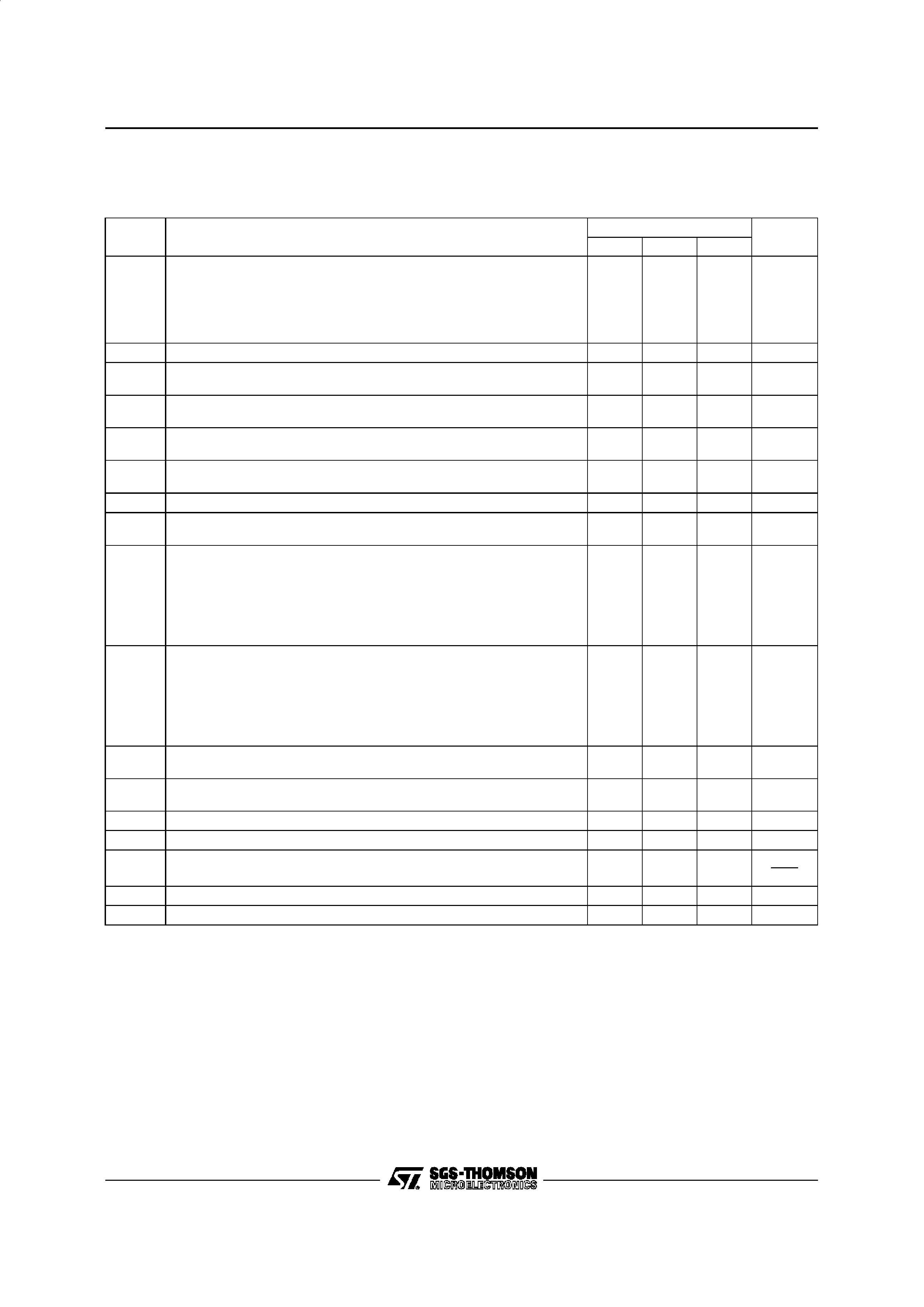ELECTRICAL CHARACTERISTICS
VCC
+ =5V, VCC- =0V, RL,CL connected to VCC/2, Tamb =25oC (unless otherwise specified)
Symbol
Parameter
TS3V912I/AI/BI
Unit
Min.
Typ.
Max.
Vio
Input Offset Voltage (Vic =Vo =VCC/2)
TS3V912
TS3V912A
TS3V912B
Tmin.
Tamb Tmax.
TS3V912
TS3V912A
TS3V912B
10
5
2
12
7
3
mV
DVio
Input Offset Voltage Drift
5
µV/oC
Iio
Input Offset Current - (note 1)
Tmin.
Tamb Tmax.
1
100
200
pA
Iib
Input Bias Current - (note 1)
Tmin.
Tamb Tmax.
1
150
300
pA
ICC
Supply Current (per amplifier, AVCL = 1, no load)
Tmin.
Tamb Tmax.
230
350
450
µA
CMR
Common Mode Rejection Ratio
Vic = 1.5 to 3.5V, Vo = 2.5V
60
85
dB
SVR
Supply Voltage Rejection Ratio (VCC
+ = 3 to 5V, VO =VCC /2)
55
80
dB
Avd
Large Signal Voltage Gain (RL = 10k
,VO = 1.5V to 3.5V)
Tmin.
Tamb Tmax.
10
7
50
V/mV
VOH
High Level Output Voltage (Vid = 1V)
RL = 100k
RL = 10k
RL = 600
RL = 100
Tmin.
Tamb Tmax.
RL = 10k
RL = 600
4.95
4.9
4.25
4.8
4.1
4.95
4.55
3.7
V
VOL
Low Level Output Voltage (Vid = -1V)
RL = 100k
RL = 10k
RL = 600
RL = 100
Tmin.
Tamb Tmax.
RL = 10k
RL = 600
40
350
1400
50
100
500
150
750
mV
Io
Output Short Circuit Current (Vid =
±1V)
Source
(Vo =VCC
-
)
Sink
(Vo =VCC
+)
45
45
65
65
mA
GBP
Gain Bandwidth Product
(AVCL = 100, RL = 10k
,CL = 100pF, f = 100kHz)
1
MHz
SR
+
Slew Rate (AVCL =1, RL = 10k
,CL = 100pF, Vi = 1V to 4V)
0.8
V/
µs
SR
-
Slew Rate (AVCL =1, RL = 10k
,CL = 100pF, Vi = 1V to 4V)
0.6
V/
µs
en
Equivalent Input Noise Voltage (Rs = 100
, f = 1kHz)
30
nV
Hz
VO1/VO2
Channel Separation (f = 1kHz)
120
dB
m
Phase Margin
30
Degrees
Note 1 : Maximum values including unavoidable inaccuracies of the industrial test.
TS3V912
4/9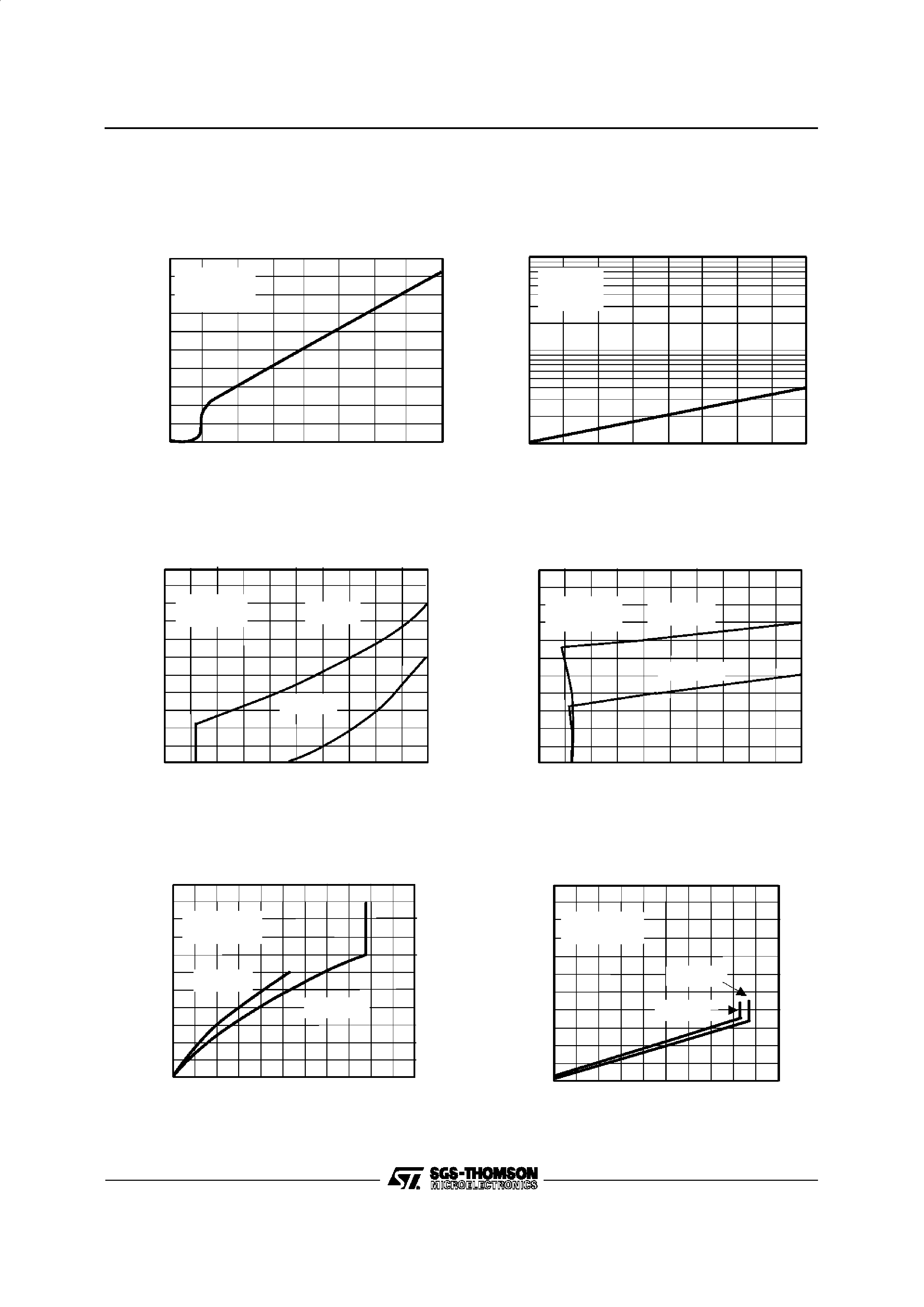TYPICAL CHARACTERISTICS
CC
S UPP LY VOLTAGE, V
(V)
04812
16
T= 25 C
A= 1
V= V
/ 2
amb
VC L
OCC
CC
µ
SUPPLY
CURRENT,
I
(
A)
600
500
400
300
200
100
Figure 1 : Supply Current (each amplifier)
versus Supply Voltage
25
50
75
10 0
125
INPUT
BIAS
CURRENT,
I
(pA)
ib
V
= 10V
V= 5V
CC
i
10 0
10
1
amb
TEMPERATURE, T
( C)
Figure 2 : Input Bias Current versus Temperature
1
14
28
42
56
70
OUTPUT
VOLTAGE,
V
(V)
OL
amb
id
T= 25 C
V
= -100mV
V
= +5V
CC
V
= +3V
CC
0
OL
OUTP UT CURRENT, I
(mA)
2
3
4
5
Figure 4a : Low Level Output Voltage versus
Low Level Output Current
2
OUTPUT
VOLTAGE,
V
(V)
OL
amb
id
T= 25 C
V
= -100mV
0
V= 1 0V
CC
V
= 16V
CC
OL
O UTPUT CURRENT, I
(mA)
4
6
8
10
14
28
42
56
70
Figure 4b : Low Level Output Voltage versus
Low Level Output Current
5
-70
-56
-42
-28
-14
0
OUTPUT
VOLTAGE,
V
(V)
OH
amb
id
T= 25 C
V
= 100mV
V
= +5V
CC
V
= +3 V
CC
4
3
2
1
0
OH
OUTPUT CURRENT, I
(mA)
Figure 3a : High Level Output Voltage versus
High Level Output Current
4
0
OUTPUT
VOLTAGE,
V
(V)
OH
V
= +16V
CC
V
= +10V
CC
OH
OUTP UT CURRENT, I
(mA)
12
8
20
16
-70
-56
-42
-28
-14
0
amb
id
T= 25 C
V
= 100mV
Figure 3b : High Level Output Voltage versus
High Level Output Current
TS3V912
5/9